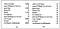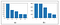# Buying a used car? Come, lets predict its price!

INTRODUCTION

MACHINE LEARNING TECHNIQUES USED

A. Multiple linear regression

Linear regression is an analytical technique that is used to determine the relationship among the different attributes used which have a reason and result relation among them. The ultimate goal of multiple regression is to relate the dependent attribute to two or more independent attributes. Technique that involve one dependent and one independent variable is called as linear regression and those that involve multiple independent variables is called as multiple regression. Multiple regression is formulated as the following

Y = β0 + β1X1 + β2X2 +…. + βnXn

Where,

Y = dependent variable

X= independent variable

β = y-intercept

The assumptions of multiple regression are the attributes considered should have a normal distribution, should be linear, should be free from the presence of outliers and should not have multiple ties among the considered independent attributes. This model has be chosen for the prediction of used car prices since the dataset has numerical values in most of its columns.

B. Random Forest regression

As the name suggests, random forest is a tree-based group where each tree is influenced by a collection of random variables. The trees employed in this technique are based on the binary periodic partitioning trees. These trees divide the dependent variable using a series of binary partitions called splits with respect to the independent variables. The root node contains the entire dependent variables. The nodes that aren’t divided are called as terminal nodes and they configure the final partition of the predictor variable. Further each non terminal node divides into two successor nodes, each in opposite directions of left and right based on the value of one of the predictor variable. Statistically, random forest has been found more appealing due to measures of variable significance, dissimilar class weighing, dealing with missing values and visualization. Also from computational point of view, random forest is more convincing since they naturally hold both regression and multiclass classification. They are also relatively quick to train and forecast. Mostly they depend on one or two tuning parameters. Another important aspect is that they can be employed in parallel and can be directly used on high-dimensional problems where the list of independent attributes is huge like the dataset I have chosen to predict the price of used cars in this paper.

DATA PREPROCESSING AND TRANSFORMATION

It’s always better to pre-process the data before we build a model. The pre-processing of the data includes checking the presence of missing values, detection of outliers if any and the univariate and bivariate analyses. The dataset that I have collected for used cars prediction consists of 31,172 rows and 20 different variables that might affect the price of the used car. Firstly, after I have imported the dataset and checked the summary as below.

The summary gives us a rough idea of our dataset including the mean, standard deviation and the quartile range values. Next, I have checked the presence of missing values in the dataset.(a) List of attributes containing missing values (b) List of attributes showing null missing values after being processed

My dataset had a number of missing values in various variables. Hence to deal with this problem, I have replaced respective missing values with values from other variables. Since the brand of car and gearbox is relatable, I have replaced gearbox missing values with respective values as per the brand column ones. Similarly, I have replaced ‘notRepairedDamage’ ones with the majority category, replaced the fuelType ones with the majority in that category, replaced missing values of vehicle type with those of the fuel type and finally the model missing variables with the majority category in that column. All these steps helped me achieve null missing values in each

independent variables column. I also wanted to remove outliers from these two columns ‘yearsofregistration’ and ‘price’. Hence I removed rows which had ‘yearsofregistration’ values before the year 1950 and after the year 2017. For the price column, I removed those below \$100 and those above \$200,000.

I have also done the univariate analysis to check if the distribution of variables counts in each variable column is normalised so that we don’t find irregular patterns in our data.(a) univariate analysis plot of fuel-type (b) univariate analysis plot of vehicle-type

Finally I have drawn the correlation heat map which helps me study the correlation coefficient of my variables. Hence I concluded on the variables that are necessary since they have high correlation and deleted other unwanted variables which might not be of any use to me while building the model.

From the correlation table, I found that all the variables I have considered for my model building are correlated to the price variable hence I have moved forward to model building from this point.

MODEL BUILDING AND EVALUATION

In this section, we are going to see the various models that have been employed in the three datasets that we obtained. The model’s performances has also been evaluated using various evaluation metrices like mean absolute error (MAE), mean squared error (MSE), r2 score, confusion matrices and ROC curves based on the algorithm that has been chosen.

The pre-processed dataset was split into parts namely X and Y parts which was further split into x_train, y_train, x_test and y_test. The X portion is the training dataset that contains all the rows and all the columns except the dependent column that needs to be predicted. Y is the test dataset portion that consists of the dependent column with all the row values. Now x_train and y_train are the parts with all the actual values with which the model learns to predict. x_test is the part on which the model applies itself and gives an prediction output. y_test is the part with actual predicted values with which the x_test predicted values are compared and evaluated for metrics like accuracy and precision etc.

As informed earlier, I have applied the linear regression and random forest techniques on this dataset since they seem apt to the dataset. The linear regression function is imported from a package called sklearn.linear_model and random forest is imported from sklearn.ensemble package. The model is built based on x_train and y_train values and predicted on the x_test values and compared with the y_test vales. The common evaluation technique that has been used for linear regression is MAE, MSE and r2 scores. MAE is the measure of errors between paired observations that indicate the same phenomenon, while MSE is the measure of the average of the squares of the errors. And r2 score is the coefficient of determination whose value varies from 0 to 1. I can also say that, it’s the ratio of variance in the dependent attributes derived from the independent attributes. When linear regression was applied to the used cars dataset, the result is as follows

Although the dataset is balanced well, I have received a r2 score of 34.2% which indicates that the model is a poor model in terms of evaluation metrics.

I have also applied random forest to the same dataset and we already know that random forest is also evaluated using the same evaluation metrics such as MSE, MAE and r2 scores. The output from application of random forest shows as

The r2 score from the random forest algorithm shows that the model is 80.3% which indicates that the model is a better model. I have also compared both the models to conclude on which model is the better one. My comparison output is as follows